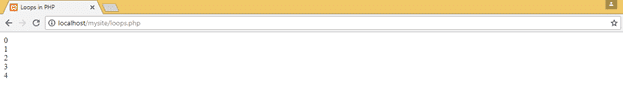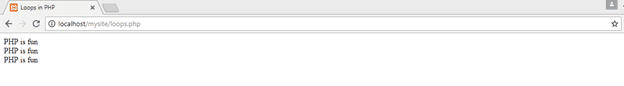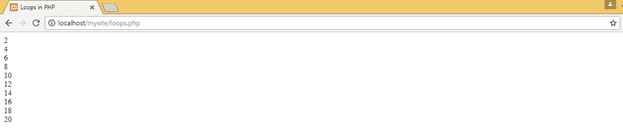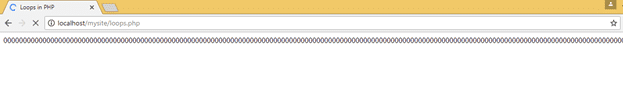## Tutorial on Types of loops in PHP

There are many kind of loops including for, while, do while and for each loop. Each one will be discussed today except for the “for each loop” Instead we will learn it with the Arrays data types. So, we use a loop when there is a need to run some code multiple times. Let’s say, we want to print “PHP is fun” 10 times on the screen. So instead of writing of 10 lines of ‘echo “PHP is fun”;’ three lines of a loop will do the job.

##### While Loop– Types of loops in PHP

It is a simple loop which has a syntax as

while(condition){

CODE;

}

This loop, will keep executing the code inside it again and again unless the condition given does not become false. Example will clear this up. (A code in a picture means that we really want you to write this with your hands instead of just copying it).Run this code and observe the output. Story starts from the very first line where we defined a variable \$i. Since variable has a value of 0 at first so condition of while becomes true and code inside it gets executed. After first execution, it will again go for while condition to check if it is still true. But now value of \$i has been incremented by 1 (because of \$i++ in the code). So it will keep checking and keep incrementing the value until \$i becomes equal to 5. After that, \$i<5 becomes false and hence the loop breaks. So, the output will look like this

#####For Loop- Types of loops in PHP

It is another form of while loop, but of great importance. We use it all the time to make our working easy and smart. As we all know three parts of while loop were really important. First was defining a variable, second was checking the condition and third was to increment in the variable. All of this syntax has been customized to ‘for loop’ this way.

For (Define a variable; Check the variable in a condition; Change in variable){

CODE;

}

Let me elaborate a little more. There are three parts inside a ‘for’ loop. First is to simply define a variable by defining a name to it and assigning a value for example. \$x=0; . This statement will only execute once.

On the second part we apply a condition for the variable defined in the first part. Lets say \$x>=10;

Third pat includes the change you want to do for the next iteration. For example x++; or x–; (we have already discussed these ++ and — operators in previous tutorials).

Here is an example of a ‘for loop’.

for(\$V=0; \$V<3; \$V++){

echo “PHP is fun <br>”;

}

Here is how it works. On first attempt it will check for the value of \$V, if it is less than 3 or not. At first value is 0. So statement will be true and hence the code inside this statement will run. On the same time, value of \$V will be increased with 1 (in part of \$V++). And again it’ll check if the value is less than 3 or not so the code will run as value will be 1 this time. It will keep executing until the value of \$V becomes equal to 3. After that, \$V<3 will become false (as 3 will be equal to \$V and not the less) and code inside the statement will not be executed this time. So a total of 3 times the Code was executed. And the final output will look like thisWe can increase the value of 3 to 10 and the code inside it will run 10 times.

##### Do While- Types of loops in PHP

Another type of loop but less famous. Syntax of this loop isIt will keep executing the code inside the do{} statement unless the condition of while becomes false. Inside the code we are multiplying 2 with the value of \$x and then printing it. Remember that it will not change the value inside the \$x because we are not saving it inside the x. We are multiplying just for the sake of printing. Actual value of \$x is changing with \$x++; statement. Output will be

#####Infinite Loop- Types of loops in PHP

Loops are really powerful but we need to use the syntax properly. Otherwise results can be drastic. One example of this situation is an infinite loop. It occurs when we use an inaccurate check condition in a loop. Or we do not increment the variable properly. So the condition will never be false and loop will continue running forever. Here is an exampleAs you can see, we are not incrementing the value of \$x anywhere. Hence it will always stuck inside the loop resulting an infinite loop.Hence this will be the output.

##### Printing A Table- Types of loops in PHP

This is a fun exercise to test your abilities and knowledge of all the previous tutorials by printing a Table of 7. Let’s start

First thing to write is the syntax of a table as we know we need something like. 7x1 = 7. Where highlighted 1 and 7 need to be changing as the table proceed. If we store ‘1’ in a variable and define another variable for the integer of which we want to print the table. We can print the whole results.

\$count=1;

\$table_Of=7;

So as we have all the variables, let’s write all the permanent parts in a string where changing parts in a variable so a combination will look like as

echo \$table_Of.”x”.\$count.” =”.(\$table_Of * \$count).”<br>”;

Now a loop is to be used to print the whole table. Let’s use a while loopAnd the output of this will make you realize the power of Variables and Loops in PHPAnd at the end, your task to do by your own is to print a table of 17 which goes from 1 to 30.That’s it for today. I hope you enjoyed coding. Let us know your review through comments.